2019/11/18 22:20

# 2. 从概率预测问题说起

## 0x1：条件概率预测模型之困

• 通过计算叶子节点上每类的相对频率，就能从决策树中得到概率
• 通过检验某条规则所覆盖的实例，就能从决策列表中得到概率

• 朴素贝叶斯分类器和Logistic回归模型只能表达简单的分布
• 决策树可以近似表达任意分布，但决策树也有缺陷，它将训练集分隔成越来越小的数据集，必然造成概率估计可靠性的下降，并且还存在重复子树问题。

• 欠拟合问题，对复杂场景的表征能力有限
• 过拟合问题，以决策树为代表的分治预测算法存在”过度编码问题“，虽然这可以通过剪枝技术得以缓解

## 0x2：解决条件概率预测的两个学术分支

• SVM（支持向量机模型）：
• SVM的决策面只和训练集中的少数实例有关，不会因为某些实例的变化而改变分界面，这从理论上证明了过拟合的发生
• SVM的决策面考虑了最大化原理类间实例距离，这提供了更强的泛化能力，缓解了欠拟合的发生
• 感知机
• 多层感知机、神经网络
• ......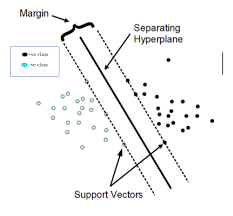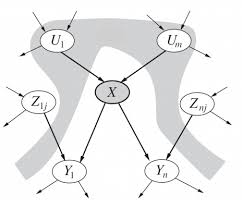## 0x3：什么场景适合贝叶斯网建模

• 整体世界观：”事物之间不是简单的二元的一对一关系，而是多对多的多元依赖关系“
• 局部世界观：”在复杂纠缠的关系网内，也存在众多的局部小关系网，这些关系网内部拥有较高的内聚性，对外保持一定的独立性。或者说整个关系网是相对稀疏的，某个事件的发生一般只和相关的某些因素有关，不太可能和世界上的所有其他因素都存在关系“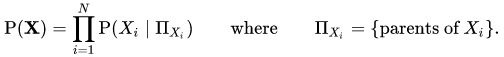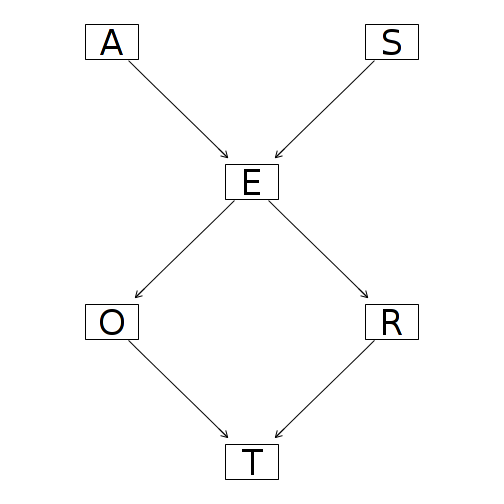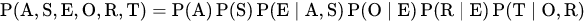《数据挖掘 实用机器学习工具与技术》Ian H.Witten Eibe Frank，Mark A.Hall

# 3. 从一个具体的例子建立贝叶斯网预测感性认识

## 0x1：一个关于天气预测的贝叶斯网预测示例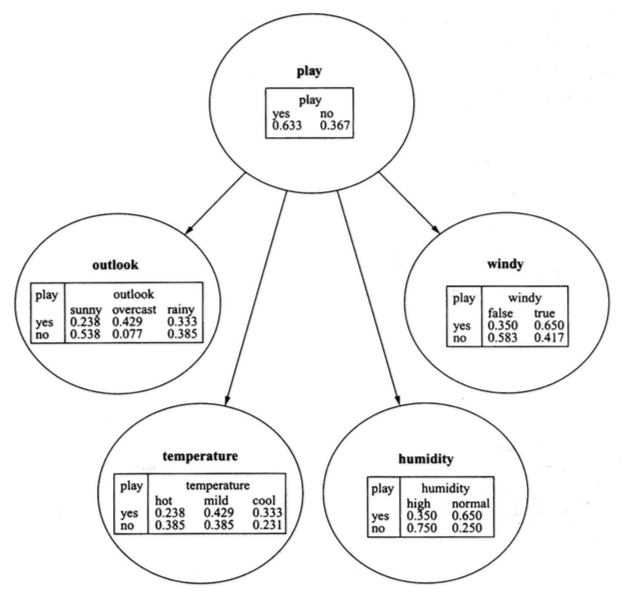• play类属性：先验概率分别为0.633和9.367，分别和4个属性结点存在一条有向边，实际上，这个贝叶斯网退化成了一个朴素贝叶斯结构
• outlook属性：在先验play的条件下，分别有：sunny、overcast、rainy这3个后验概率属性
• windy属性：在先验play的条件下，分别有：false、true这2个后验概率属性
• temperature属性：在先验play的条件下，分别有：hot、mild、cool这3个后验概率属性
• humidity属性：在先验play的条件下，分别有：high、normal这2个后验概率属性

• 网络结构：结点间的连接方式，结点间的有向线段代表相关关系，并不一定是因果关系
• 结点属性的概率值：结点内部属性的条件概率分布，每行的概率对应于一组父节点属性值的组合，行中的每个概率代表了这个结点属性的每个属性值对应于该属性值组合的概率。每行中各个概率的综合始终为1

## 0x2：一个稍微复杂一些的天气预测例子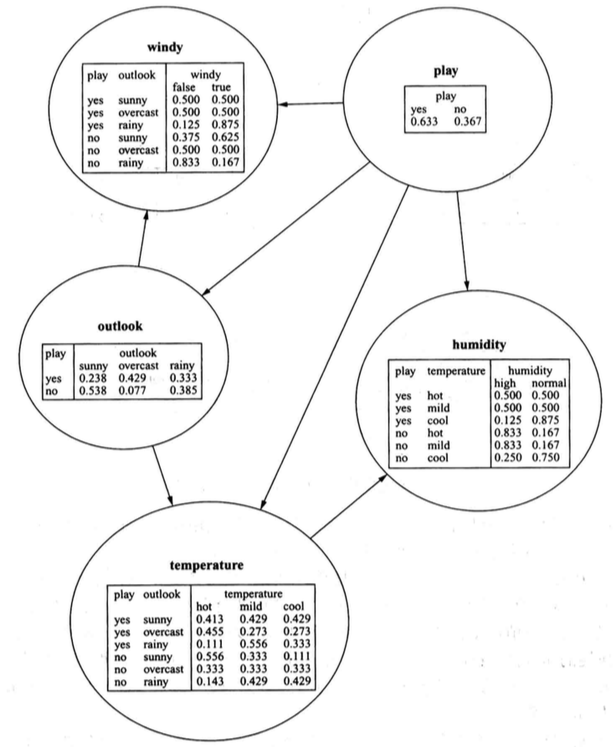### 1. 基于贝叶斯概率链式法则计算实例每个类值概率

• P（play=no）= 0.367
• P（outlook=rainy | play=no）= 0.385
• P（temperature=cool | play=no，outlook=rainy）= 0.429
• P（humidity=high | play=no，temperature=cool）= 0.250
• P（windy=true | play=no，outlook=rainy）= 0.167

• P（play=no，outlook=rainy，temperature=cool，humidity=high，windy=true）=
• P（play=no）*
• P（windy=true | play=no，outlook=rainy）
• P（outlook=rainy | play=no）*
• P（temperature=cool | play=no，outlook=rainy）*
• P（humidity=high | play=no，temperature=cool）*
• = 0.367 * 0.385 * 0.429 * 0.250 * 0.167 = 0.00253069942125
• 同理有：P（play=yes，outlook=rainy，temperature=cool，humidity=high，windy=true）= 0.0077

【play=？outlook=overcast，temperature=cool，humidity=high，windy=true】

outlook属性值的不同，使概率链条进入了另一个分布空间

### 2. 贝叶斯网概率计算中链式分解法则的解释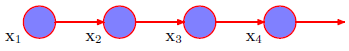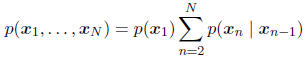• P(play=?，windy=?，outlook=?，humidity=?，temperature=?) =
• P(play)
• P(windy | play)
• P(outlook | windy，play) *
• P(temperature | outlook，windy，play) *
• P(humidity | temperature，toutlook，windy，play) *

• P(play=?，windy=?，outlook=?，humidity=?，temperature=?) =
• P(play) = P(play)
• P(windy | play) = P(windy | play，outlook)
• P(outlook | windy，play) = P(outlook | play)
• P(temperature | toutlook，windy，play) = P(temperature | outlook，play)
• P(humidity | temperature，toutlook，windy，play) = P(humidity | temperature，play)

• P（play=no，outlook=rainy，temperature=cool，humidity=high，windy=true）=
• P（play=no）*
• P（windy=true | play=no，outlook=rainy）
• P（outlook=rainy | play=no）*
• P（temperature=cool | play=no，outlook=rainy）*
• P（humidity=high | play=no，temperature=cool）*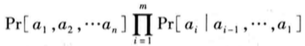a1, a2,.. 代表图中每个节点，Par(ai)就表示除了ai以外的所有节点。公式中的逗号表示“与”的关系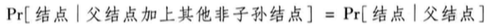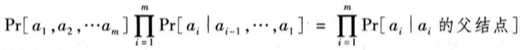### 3. 两种贝叶斯网络的不同

• 第一个网络具有更严格的独立假设，它的每个结点的父节点都是实例类属性结点。实际上，它就是朴素贝叶斯分类器
• 第二个网络中的条件概率列表含有更多的行，因此网络需要更多的参数。它可以对潜在领域进行更精确地描述

## 0x3：警惕因果推断陷阱

https://www.cnblogs.com/LittleHann/p/11683607.html#_label3_2_1_1

# 4. 贝叶斯网训练

• 网络结点连接结构：一个有向无环图（DAG），其中每个结点 vi ∈ V 对应一个随机变量 Xi
• 结点属性内的条件概率分布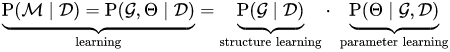## 0x1：贝叶斯网结点结构学习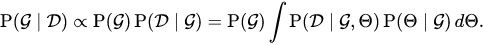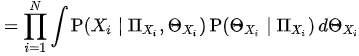• “网络结构质量评估方法”：基于局部条件独立性进行概率累计运算
• “网络拓朴空间搜索方法”：如何在指数级的穷举空间中有效地进行拓朴搜索

### 1. 基于某个数据集的网络结构质量评估函数

• 采用交叉验证来估计拟合的良好度
• 增加对网络参数数量的惩罚项
• 赋予网络结构一个先验分布，通过组合先验概率以及数据与网络相符的概率，找出可能性最大的网络。这是网络评分的“贝叶斯”方法

• Akaike信息准则（Akaike Information Criterion，AIC）：AIC得分 = -LL + K
• 其中LL表示概率对数似然结果
• K为参数数量
• 基于最短描述长度原理的MDL度量：MDL得分 = -LL + K/2 * logN
• 其中N为数据集中的实例数量

### 2. 网络结点有向边连接空间搜索策略

#### 1）K2算法

K2算法是一个简单而快速的启发式算法，简要描述如下：

• 算法起始于某个给定的属性（即结点）的排序
• 然后对每个结点依次进行处理，
• 贪心地增加从先前处理过的结点指向当前结点的边
• 每一步过程中都增加那些能使网络得分达到最高值的边
• 当不再有改进时，便将注意力转向下一个结点
• 每个结点的父节点数量可以限制在一个预设的最大值范围内，这是为防止过度拟合附加的机制

#### 5）HHMC启发式搜索算法

https://www.google.com.hk/search?newwindow=1&safe=strict&hl=zh-CN&sxsrf=ACYBGNQ6fvIClIfRwRyXUasIvDRpeZpjEQ%3A1574044570225&source=hp&ei=mgPSXYb0Cs6AtgX18K6QBg&q=mmhc.r+&oq=mmhc.r+&gs_l=psy-ab.3...1738.1738..1925...0.0..0.0.0.......0....2j1..gws-wiz.&ved=0ahUKEwiGqd373PLlAhVOgK0KHXW4C2IQ4dUDCAY&uact=5

## 0x2：贝叶斯网结点条件概率学习《数据挖掘 实用机器学习工具与技术》Ian H.Witten Eibe Frank，Mark A.Hall

# 5. 贝叶斯网推断（Bayesnetwork Inference）

• 贝叶斯网最大后验概率估计推断（Bayesnetwork Maximum Posteriori (MAP)  Inference）：从一个已知证据出发，推断其他未知因素的出现概率
• 贝叶斯网条件概率查询（Bayesnetwork Conditional Probability Queries）：已知一个实例，推断其在该贝叶斯网条件下出现的似然概率

## 0x1：贝叶斯网最大后验概率估计推断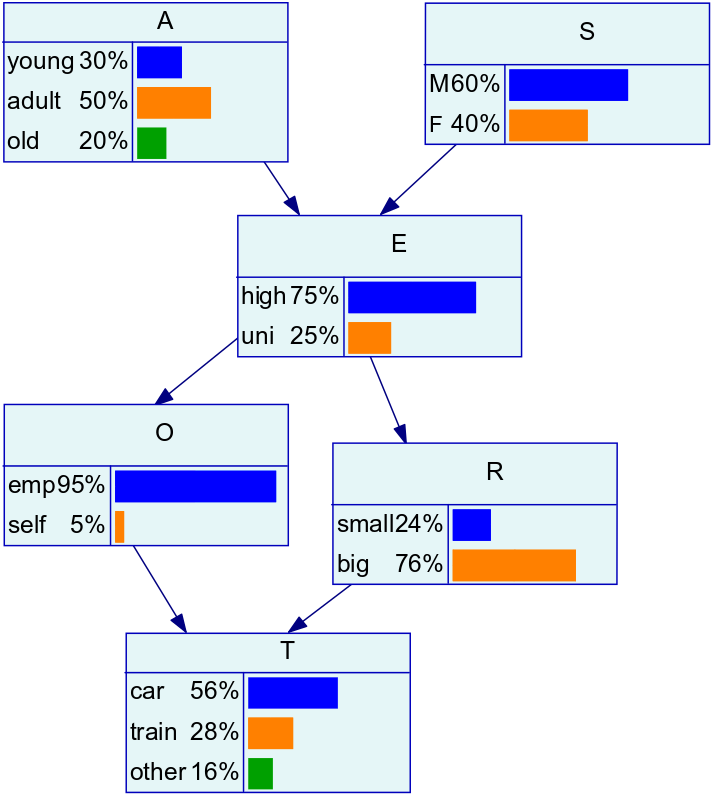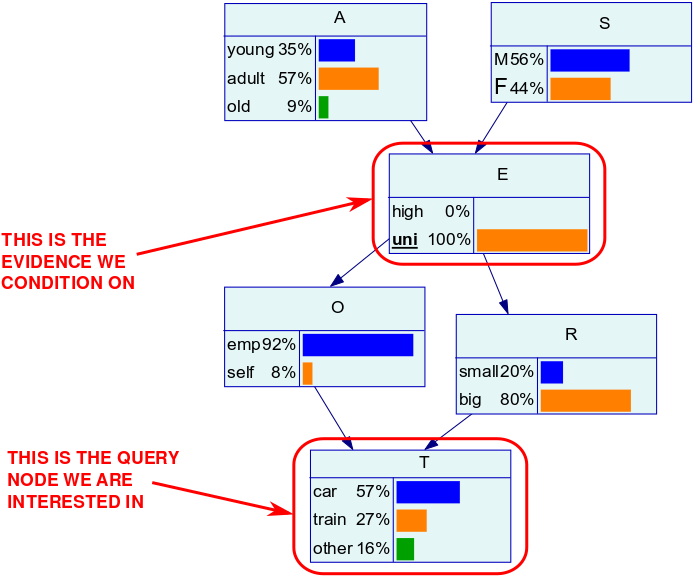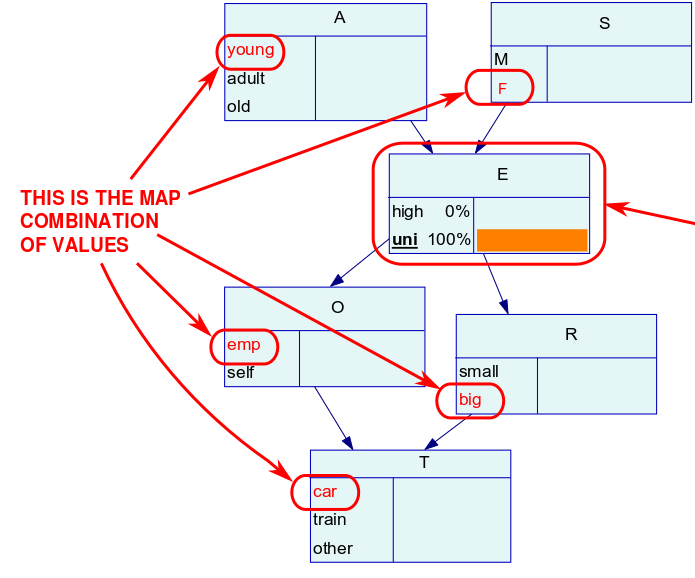# 6. pomegranate - 一种高效的概率图建模框架

## 0x1：基本使用介绍

### 1. 从已知模型参数中创建概率分布

# -*- coding:utf-8 -*-

from pomegranate import *
import pygraphviz as pgv
import matplotlib.pyplot as plt

def plot_xy(x, y):
plt.plot(x, y)
plt.show()

if __name__ == '__main__':
mu, sig = 0, 2
model = NormalDistribution(mu, sig)

x_y = {}
for i in range(9999):
x = float("%.1f" % model.sample())
if x_y.has_key(x):
x_y[x] += 1
else:
x_y[x] = 1

x_y = sorted(x_y.items(), key=lambda d: d)
print x_y

x = [i for i in x_y]
y = [i for i in x_y]

print x
print y

plot_xy(x, y)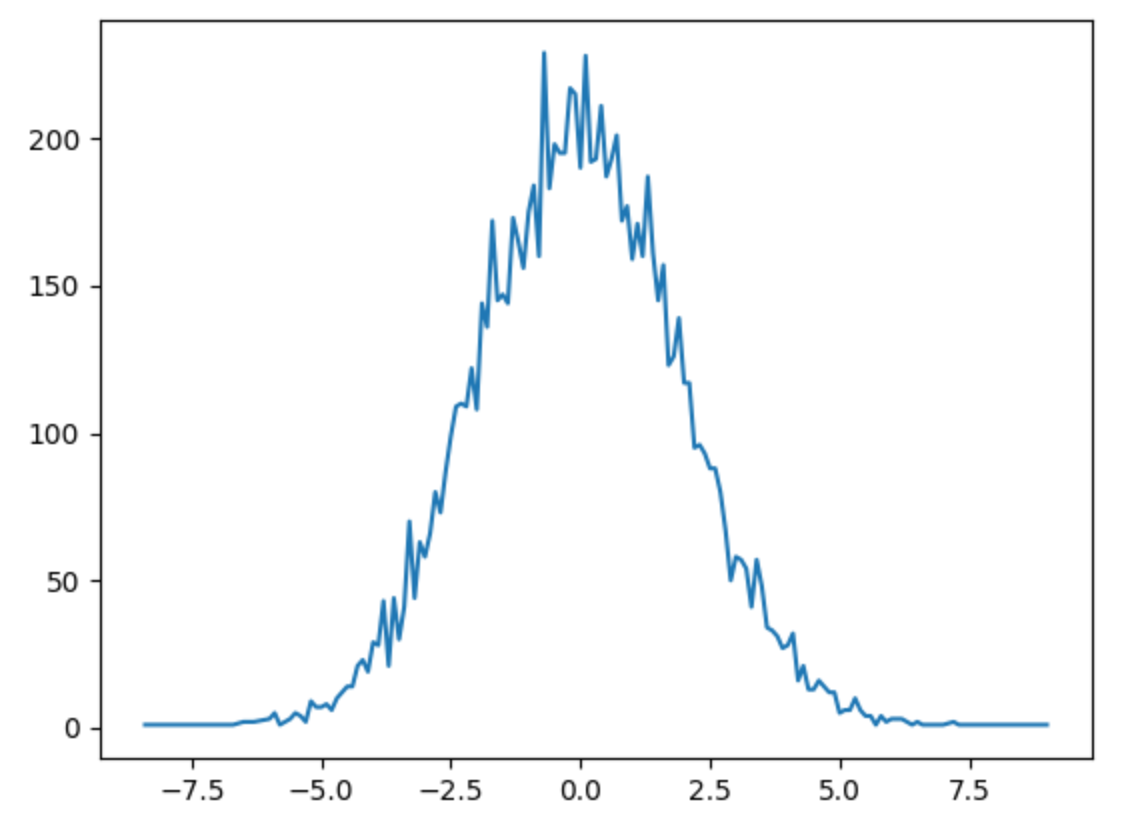### 2. 从数据中直接学习概率分布

# -*- coding:utf-8 -*-

from pomegranate import *
import pygraphviz as pgv
import matplotlib.pyplot as plt
import numpy as np

def plot_xy(x, y):
plt.plot(x, y)
plt.show()

def generate_data():
X = np.random.normal(0, 1, 100)
model = NormalDistribution.from_samples(X)

return model

def generate_xy_plot(model):
x_y = {}
for i in range(9999):
x = float("%.1f" % model.sample())
if x_y.has_key(x):
x_y[x] += 1
else:
x_y[x] = 1

x_y = sorted(x_y.items(), key=lambda d: d)
print x_y

x = [i for i in x_y]
y = [i for i in x_y]

print x
print y

return x,y

if __name__ == '__main__':
model = generate_data()
x, y = generate_xy_plot(model)

plot_xy(x, y)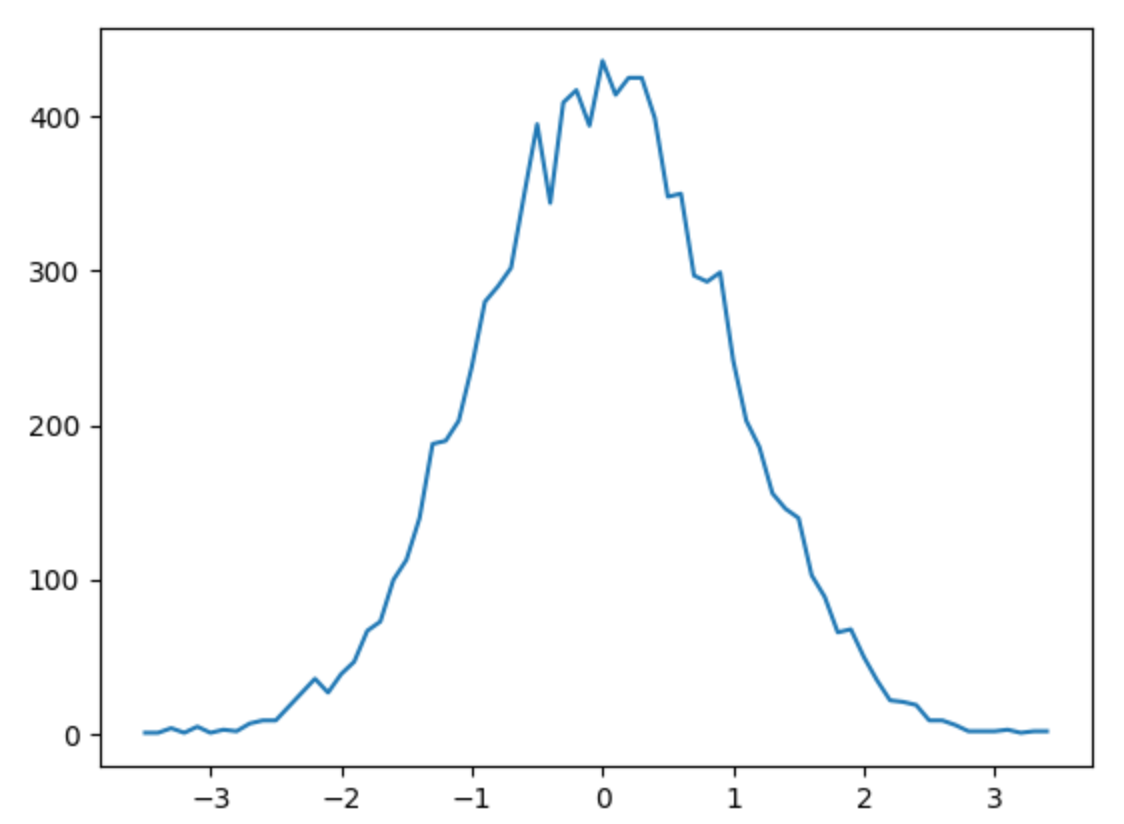### 3. HMM detection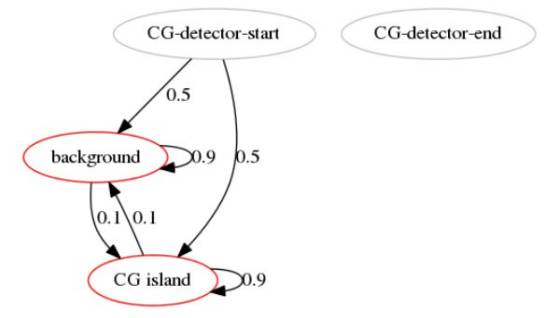# -*- coding:utf-8 -*-

from pomegranate import *
import pygraphviz as pgv
import matplotlib.pyplot as plt
import numpy as np

if __name__ == '__main__':
d1 = DiscreteDistribution({
'A': 0.25,
'C': 0.25,
'G': 0.25,
'T': 0.25
})
d2 = DiscreteDistribution({
'A': 0.10,
'C': 0.40,
'G': 0.40,
'T': 0.10
})

s1 = State(d1, name="background")   # 隐状态
s2 = State(d2, name="CG island")    # 显状态

hmm = HiddenMarkovModel("CG-detector")

# 状态概率转移矩阵

# 隐状态和显状态的概率发射矩阵
hmm.bake()

#
observed_sequence = "GACTACGACTCGCGCTCGCGCGACGCGCTCGACATCATCGACACGACACTC"

print "how likely the sequence happen: ", hmm.log_probability(list(observed_sequence))
print "the corresponding hidden state sequence: ", hmm.predict(list(observed_sequence))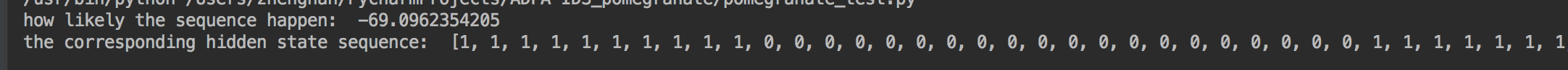### 4. 贝叶斯网建模”Monty Hall problem“

• 参赛选手的选择
• 奖品所在的门
• 主持人打开的门

# -*- coding:utf-8 -*-

from pomegranate import *
import pygraphviz as pgv
import matplotlib.pyplot as plt
import numpy as np

if __name__ == '__main__':
guest = DiscreteDistribution({'A': 1. / 3, 'B': 1. / 3, 'C': 1. / 3})
prize = DiscreteDistribution({'A': 1. / 3, 'B': 1. / 3, 'C': 1. / 3})
monty = ConditionalProbabilityTable(
[['A', 'A', 'A', 0.0],
['A', 'A', 'B', 0.5],
['A', 'A', 'C', 0.5],
['A', 'B', 'A', 0.0],
['A', 'B', 'B', 0.0],
['A', 'B', 'C', 1.0],
['A', 'C', 'A', 0.0],
['A', 'C', 'B', 1.0],
['A', 'C', 'C', 0.0],
['B', 'A', 'A', 0.0],
['B', 'A', 'B', 0.0],
['B', 'A', 'C', 1.0],
['B', 'B', 'A', 0.5],
['B', 'B', 'B', 0.0],
['B', 'B', 'C', 0.5],
['B', 'C', 'A', 1.0],
['B', 'C', 'B', 0.0],
['B', 'C', 'C', 0.0],
['C', 'A', 'A', 0.0],
['C', 'A', 'B', 1.0],
['C', 'A', 'C', 0.0],
['C', 'B', 'A', 1.0],
['C', 'B', 'B', 0.0],
['C', 'B', 'C', 0.0],
['C', 'C', 'A', 0.5],
['C', 'C', 'B', 0.5],
['C', 'C', 'C', 0.0]], [guest, prize])

s1 = Node(guest, name="guest")
s2 = Node(prize, name="prize")
s3 = Node(monty, name="monty")

model = BayesianNetwork("Monty Hall Problem")
model.bake()

model.plot()

### 5. 贝叶斯网问题预测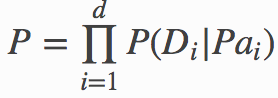• Guest选择门编号的条件概率
• 奖品所在门编号的概率
• 主持人Monty在看到Guest作出选择后，所打开门编号的概率
# -*- coding:utf-8 -*-

from pomegranate import *
import pygraphviz as pgv
import matplotlib.pyplot as plt
import numpy as np

if __name__ == '__main__':
guest = DiscreteDistribution({'A': 1. / 3, 'B': 1. / 3, 'C': 1. / 3})
prize = DiscreteDistribution({'A': 1. / 3, 'B': 1. / 3, 'C': 1. / 3})
monty = ConditionalProbabilityTable(
[['A', 'A', 'A', 0.0],
['A', 'A', 'B', 0.5],
['A', 'A', 'C', 0.5],
['A', 'B', 'A', 0.0],
['A', 'B', 'B', 0.0],
['A', 'B', 'C', 1.0],
['A', 'C', 'A', 0.0],
['A', 'C', 'B', 1.0],
['A', 'C', 'C', 0.0],
['B', 'A', 'A', 0.0],
['B', 'A', 'B', 0.0],
['B', 'A', 'C', 1.0],
['B', 'B', 'A', 0.5],
['B', 'B', 'B', 0.0],
['B', 'B', 'C', 0.5],
['B', 'C', 'A', 1.0],
['B', 'C', 'B', 0.0],
['B', 'C', 'C', 0.0],
['C', 'A', 'A', 0.0],
['C', 'A', 'B', 1.0],
['C', 'A', 'C', 0.0],
['C', 'B', 'A', 1.0],
['C', 'B', 'B', 0.0],
['C', 'B', 'C', 0.0],
['C', 'C', 'A', 0.5],
['C', 'C', 'B', 0.5],
['C', 'C', 'C', 0.0]], [guest, prize])

s1 = Node(guest, name="guest")
s2 = Node(prize, name="prize")
s3 = Node(monty, name="monty")

model = BayesianNetwork("Monty Hall Problem")
model.bake()

print model.probability([['A', 'A', 'A'],
['A', 'A', 'B'],
['C', 'C', 'B']])https://www.ctolib.com/jmschrei-pomegranate.html
https://blog.laisky.com/p/pygraphviz/
http://www.sohu.com/a/150274125_505915
https://github.com/jmschrei/pomegranate/blob/master/docs/BayesianNetwork.rst
https://pomegranate.readthedocs.io/en/latest/BayesianNetwork.html

# 7. bnlearn

http://www.bnlearn.com/examples/
http://www.bnlearn.com/examples/useR19-tutorial/
http://www.bnlearn.com/book-crc/

0
0 收藏

### 作者的其它热门文章0 评论
0 收藏
0